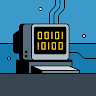# C Program to find the number of digits in an integer## Finding the number of digits in an integer in C

Today, we will be learning how to find the number of digits in an integer in C . An integer is made up of a group of digits, i.e; it is a combination of digits from 0-9

Here we will use loops along with an arithmetic operator.This program takes an integer from the user and calculates the number of digits. For example: If the user enters 6589, the output of the program will be 4.

## Method Discussed :

• Method 1 : Using loop
• Method 2 : Using formulae.

Let’s discuss above two methods in brief,

## Method 1 :

• Take a variable count =  0, to store the count of digits in the given number.
• Run a while loop till n>2
• Inside the loop increment the value of count by 1
• Set n=n/10
• After complete iteration print the value of count.

### Method 1 : Code in C

Run
```#include <stdio.h>
#include <math.h>

int main(){
int n = 20901;
int count = 0;

while(n>0){
count++;
n = n/10;
}
printf("No. of digits = %d", count);

}
```

### Output

```No. of digits = 5
```

## Method 2 :

In this method we will simple use the mathematical formulae,

• No. of digits : floor(log10(n))+1

### Method 2 : Code in C

Run
```#include<stdio.h>
#include<math.h>

int main(){
int n = 20901;
int x = floor(log10(n))+1;

printf("No. of digits = %d", x);

}
```

### Output

```No. of digits = 5
```

### One comment on “C Program to find the number of digits in an integer”

•Abhishek

*SIMPLE FORM IN C
#include
void main()
{
int n,rem=0,count=1;
printf(“Enter the input”);
scanf(“%d”,&n);
while(n>0)
{
rem=n%10;
n=n/10;
rem=count++;
}
printf(“Number of digit in a number is: %d”,rem);
}2